You are here
Home > Class 11 > Straight Lines class 11 Chapter 10

# Straight Lines class 11 Chapter 10## Straight Lines## What is a Straight Line?

Lines can be parallel, perpendicular, intersecting, or concurrent. A straight line is the set of all points between and extending beyond two points.  In Euclidean geometry, the two properties of straight lines are that they have only one dimension, length, and they forever continue in two directions.

## Different Types of Formula

• ### Distance formula

Distance between (x1,y1) and (x2,y2) is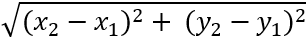• ### Section Formula

i) Internal Divisionii) External Division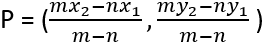Mid point =• ### Area of a triangle

(x1,y1) , (x2,y2) and (x3,y3) are three vertices of a triangle

Area =[x1(y2-y3) + x2(y3-y1)+ x3(y1 – y2)]

• ### Slope of a line

Slope , m = tanθ where θ is the angle which the line makes with positive direction of X- axis in anti clockwise direction [00 ≤ θ≤ 1800]

m =slope of X-axis = 0

slope of Y-axis = ∞

If the lines are parallel, m1 = m2

If the lines are perpendicular m1.m2 = -1

## Problems Related to Straight lines

Q. Slope of a line is 5. Write the slope of another line parallel to the give line

5

Q. Slope of a line is 2/3. Write the slope of another line perpendicular to the give line

– 3/2

Q. Find the angle between X axis and the line joining (3,-1) and (4,-2)

m == (-2+1)/(4-3) = -1

Tanθ = -1

θ= 1800 – 450 = 1350

Q. Find the value of x for which the points (x,-1),(2,1) and (4,5) are collinear

Slope of AB =Slope of BC == 2

2/(2-x) = 2

2 = 2(2-x)

1= 2-x

x=1

Q. Find the slope of a line which is equally inclined to the axis

θ= 00 or 1350

m = tan θ = tan 0 = 0

m= tan θ = tan 1350 = -1

Different forms of equations of a straight line

Equation of X- axis : y = 0,Equation of Y- axis : x = 0

Equation of a line parallel to X-axis : y =K,Equation of a line parallel to Y-axis : x =K

Point slope form

The equation of a line passing through (x1,y1) and having slope m is

y– y1 = m(x – x1)

This form is called point slope form

Q. Find the equation of a line passing through (-3,5) and perpendicular to the line through (2,5) and (-3,6)

Slope of the given line=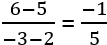Slope of the required line is 5 and passing through (-3,5)

y– y1 = m(x – x1)

y– 5 = 5(x +3)

y-5 = 5x+15

5x-y+20 = 0

Q. Find the equation of the right bisector of the line segment joining A(3,4) and B(-1,2)

C is the mid point of AB

C =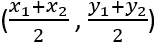=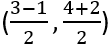= (1,3)

Slope of AB = (2-4)/(-1-3) = (-2)/(-4) = 1/2

The slpoe of PQ is -2 and passing through (1.3)

y– y1 = m(x – x1)

y– 3 =-2 (x – 1)

y-3 = -2x+2

2x+y-5 = 0

Q. The vertices of a triangle are A(2,3), B(4,-1) and C(1,2). Find the equation of the altitude from A

Slope of BC = (2+1)/(1-4) = -1

Slope of the altitude AD is 1 and passing through A(2,3)

y– 3 = 1(x – 2)

y-3 = x-2

x-y+1 = 0

Two point form

The equation of a line passing through (x1,y1) and (x2,y2) is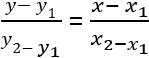Q. The vertices of a triangle PQR are P(2,1), Q(-2,3) and R(4,5). Find the equation of median through R

The mid pointof PQ, R == (0,2)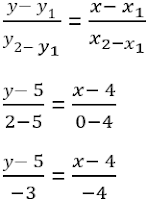(y- y_1)/y_(2- y_1 )  = (x- x_1)/x_(2-x_1 )

or, (y- 5)/(2-5) = (x- 4)/(0-4)

(y- 5)/(-3) = (x- 4)/(-4)

4(y-5) = 3(x-4)

4y -20 = 3x – 12

3x-4y+8 = 0

Q. By using the concept of equation of a line show that the points (3,0),(-2,-2) and (8,2)are collinear

The equation of the line joining (3,0) and (-2,-2) is

(y- y_1)/y_(2- y_1 )  = (x- x_1)/x_(2-x_1 )

(y-0)/(-2-0) = (x-3)/(-2-3)

(y-0)/(-2) = (x-3)/(-5)

5y = 2(x-3)

5y = 2x-6

2x-5y -6 =0

Put x = 8 and y = 2

2.8 -5.2 -6 = 0

16-10-6 = 0

0=0

(8,2) is a point in the line

The points are collinear

Intercept form

The intercept form of a line is

x/a + y/b = 1

a is the x intercept and b is the y intercept of the line

Q. find the equation of a line which makes equal intercepts on the axes and passing through (2,3)

Let x intercept = a

y intercept = a

x/a + y/a = 1

(x+y)/a = 1

x+y =a

The line passing through (2,3)

2+3 = a

a = 5

x+y = 5

Q. find the equation of a line passing through (2,2) and cutting off intercepts on the axes whose sum is 9

Let x intercept = a

Y intercept = 9 – a

x/a + y/(9-a) = 1

(x(9-a)+ya)/(a(9-a)) = 1

x(9-a) + ya = a(9-a)

Put x = 2, y = 2

2(9-a) +2a = a(9-a)

18-2a+2a = 9a – a2

a2-9a+18 = 0

(a-6)(a-3) = 0

a=6,3

Case 1

a=6

b=3

x/a + y/b = 1

x/6 + y/3 = 1

Case 2

a=3

b=6

x/3 + y/6 = 1

Q. Find the equation of a line such that the midpoint of the line segment between the axes is (a,b)

X intercept = 2a

Y intercept = 2b

x/2a + y/2b = 1

x/a + y/b = 2

Slope intercept form

y = mx + c is the slope intercept form of a line where m is the slope and c is the y intercept

Normal form

x cosω + y sinω = p is the normal form. P is the length of the perpendicular from the origin to the line and ω is the angle which the perpendicular makes with positive direction of x-axis

Find a notes for Straight lines